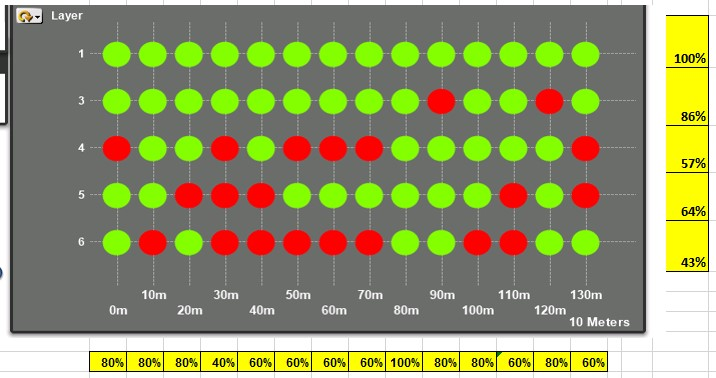# QlikView App Dev

Discussion Board for collaboration related to QlikView App Development.

Announcements
Skip the ticket, Chat with Qlik Support instead for instant assistance.
cancel
Showing results for
Did you mean:Not applicable

## Percent Totals for Grids

Hi I have Grid chart as shown below where the average temperature of a process is being recorded for every layer (1 to 6) and every 10m (0 to 130m). To achieve this the average value (Avg(\$(vFieldChart1Ring1)) is calculated by Layer (Y) and distance (X) , in this case the distance is every 10m, but I also group the data into 250mm, 500mm & 1m.What I would like to do is show the total percentages as highlighted in Yellow above both both Layers and distance.

To achieve the Red and Green Colours I have an expression under the background colours where the average value is compared to variables representing upper and lower limits.

vGridR1LCL = Lower Limit

vGridR1UCL = Upper Limit

=if(avg(\$(vFieldChart1Ring1))>= vGridR1LCL, if(avg(\$(vFieldChart1Ring1))<= vGridR1UCL, RGB(128,255,0),RGB(255,0,0)),RGB(255,0,0)) // Green Red, Red

I Have then used the same expression on a Straight Table but replaced the Green with 1 and the Red with 0 to display 100% or 0% against every dot.

=if(avg(\$(vFieldChart1Ring1))>= vGridR1LCL, if(avg(\$(vFieldChart1Ring1))<= vGridR1UCL, 1,0),0).

My problem comes when I try to group the data by both X & Y (Distance & Layer) to show the percentage total’s as highlighted in Yellow.

I’ve tried using the “Aggr”  function to group the data and show the results

Group R1 Test Totals Test 1:- This just give 100% for every distance group.

=Aggr(if(avg(\$(vFieldChart1Ring1))>= vGridR1LCL, if(avg(\$(vFieldChart1Ring1))<= vGridR1UCL, 1,0),0),([XPosition R1 10m]/1000))

Group R1 Test Totals Test 2:- This just give 100% for every distance group.

=Sum(AGGr(if(avg(\$(vFieldChart1Ring1))>= vGridR1LCL, if(avg(\$(vFieldChart1Ring1))<= vGridR1UCL, 1,0),0),([XPosition R1 10m]/1000),[R1 Layer]))Any help would be appreciated.

Thanks

Mark

1 Solution

Accepted SolutionsNot applicable
Author

If any one is interested here is the answer to having the total percentages in the horizontal line. Works fine except it takes a long time to calculate.

aggr(sum(aggr(if(avg(\$(vFieldChart1Ring1))>= vGridR1LCL, if(avg(\$(vFieldChart1Ring1))<= vGridR1UCL, 1,0),0), (\$(vGridXpos1)),(\$(vGridXpos2)))), (\$(vGridXpos1))) / Aggr(Count(DISTINCT (\$(vGridXpos2)) ), (\$(vGridXpos1)))

// vGridXpos1 = Allocates a number based apon Dist Group and Ring 1 or Ring 2 using the Match command

// vGridXpos2 = Determine's the field  in the Cylic Group Layer or Layer and Pass for Ring 1 or Ring 2Not applicable
Author

If any one is interested here is the answer to having the total percentages in the horizontal line. Works fine except it takes a long time to calculate.

aggr(sum(aggr(if(avg(\$(vFieldChart1Ring1))>= vGridR1LCL, if(avg(\$(vFieldChart1Ring1))<= vGridR1UCL, 1,0),0), (\$(vGridXpos1)),(\$(vGridXpos2)))), (\$(vGridXpos1))) / Aggr(Count(DISTINCT (\$(vGridXpos2)) ), (\$(vGridXpos1)))

// vGridXpos1 = Allocates a number based apon Dist Group and Ring 1 or Ring 2 using the Match command

// vGridXpos2 = Determine's the field  in the Cylic Group Layer or Layer and Pass for Ring 1 or Ring 2Community Browser